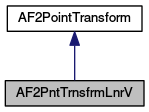MeshKit  1.0
AF2PntTrnsfrmLnrV Class Reference

`#include <AF2PntTrnsfrmLnrV.hpp>`

Inheritance diagram for AF2PntTrnsfrmLnrV:[legend]

List of all members.

## Public Member Functions

AF2PntTrnsfrmLnrV (std::list< const AF2RuleExistVertex * > vertices, std::list< double > xDiffCoeff, std::list< double > yDiffCoeff)
Constructor with lists.
AF2PntTrnsfrmLnrV (std::vector< const AF2RuleExistVertex * > vertices, std::vector< double > xDiffCoeff, std::vector< double > yDiffCoeff)
Constructor with vectors.
virtual AF2PntTrnsfrmLnrVclone () const
Makes and returns an independent copy of this AF2PntTrnsfrmLnrV.
virtual AF2Point2D transformPoint (AF2Point2D const &point, AF2Binding const &vBinding) const
Implements AF2PointTransform::transformPoint.

## Private Attributes

std::vector< const
AF2RuleExistVertex * >
refVertices
std::vector< double > xCoeff
std::vector< double > yCoeff

## Detailed Description

Definition at line 25 of file AF2PntTrnsfrmLnrV.hpp.

## Constructor & Destructor Documentation

 AF2PntTrnsfrmLnrV ( std::list< const AF2RuleExistVertex * > vertices, std::list< double > xDiffCoeff, std::list< double > yDiffCoeff )

Constructor with lists.

Construct an AF2PntTrnsfrmLnrV that is based on the differences between bound locations of the specified vertices and transforms these differences using a linear transformation with the specified coefficients.

The lists of coefficients should be twice as long as the list of vertices. For the vertex v at position i in the list of vertices (where the first position is i = 0), the xDiffCoeff at position 2*i defines the coefficient on the contribution of x-coordinate difference for vertex v to the x translation, and the xDiffCoeff at position 1 + 2*i defines the coefficient on the contribution of the y-coordinate difference for vertex v to the x translation. Similarly, the yDiffCoeff at positions 2*i and 1 + 2*i define the coefficients of the contributions of the x and y-coordinate differences for vertex v to the y translation.

For example, if a single vertex v is given the xDiffCoeff are 0.5, 0, and the yDiffCoeff are 0, 0, then the transformation will translate a point by half of the difference between the x-coordinate of the vertex to which v is bound and the x-coordinate of the reference location of vertex v.

If the coefficient lists are not exactly twice as long as the list of vertices, this method will throw an exception.

This object does not take ownership of the AF2RuleExistVertex that are passed into the constructor. It is the responsibility of the context to ensure that the pointers remain valid as long as this object or a copy, assignment, or clone of it may be used to transform a point.

Parameters:
 vertices a list of AF2RuleExistVertex xDiffCoeff a list of double values twice as long as the list of vertices that defines coefficients affecting translation in the x direction yDiffCoeff a list of double values twice as long as the list of vertices that defines coefficients affecting translation in the y direction

Definition at line 10 of file AF2PntTrnsfrmLnrV.cpp.

 AF2PntTrnsfrmLnrV ( std::vector< const AF2RuleExistVertex * > vertices, std::vector< double > xDiffCoeff, std::vector< double > yDiffCoeff )

Constructor with vectors.

This constructor is equivalent to the constructor with lists, but allows passing in vectors instead. See the constructor with lists for additional documentation.

Parameters:
 vertices a vector of AF2RuleExistVertex xDiffCoeff a vector of double values twice as long as the vector of vertices that defines coefficients affecting translation in the x direction yDiffCoeff a vector of double values twice as long as the vector of vertices that defines coefficients affecting translation in the y direction

Definition at line 39 of file AF2PntTrnsfrmLnrV.cpp.

## Member Function Documentation

 AF2PntTrnsfrmLnrV * clone ( ) const` [virtual]`

Makes and returns an independent copy of this AF2PntTrnsfrmLnrV.

Implements AF2PointTransform::clone. See additional documentation there.

Implements AF2PointTransform.

Definition at line 66 of file AF2PntTrnsfrmLnrV.cpp.

 AF2Point2D transformPoint ( AF2Point2D const & point, AF2Binding const & vBinding ) const` [virtual]`

Implements AF2PointTransform::transformPoint.

See the documentation at AF2PointTransform::transformPoint for the general contract for this method.

This implementation consults the binding and computes the differences between the actual bound location and reference location of certain vertices. The point is then translated from its current position by a vector that is the result of a linear transformation applied to the differences.

If one of the vertices that this transformation is supposed to use is not bound to a value in the binding, this method will throw an exception.

Parameters:
 point the coordinates of some 2-dimensional point vBinding a binding of reference vertices and edges to points with actual coordinates and edges between those points
Returns:
a point offset from the original point determined by a linear transformation and the bound locations of certain vertices

Implements AF2PointTransform.

Definition at line 71 of file AF2PntTrnsfrmLnrV.cpp.

## Member Data Documentation

 std::vector refVertices` [private]`

Definition at line 29 of file AF2PntTrnsfrmLnrV.hpp.

 std::vector xCoeff` [private]`

Definition at line 30 of file AF2PntTrnsfrmLnrV.hpp.

 std::vector yCoeff` [private]`

Definition at line 31 of file AF2PntTrnsfrmLnrV.hpp.

The documentation for this class was generated from the following files: